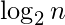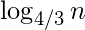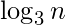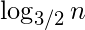Exercise: Questions on Binary Trees

Questions on Binary Trees : Question 1 :
Which of the following is a true about Binary Trees

 Every binary tree is either complete or full. Every complete binary tree is also a full binary tree. Every full binary tree is also a complete binary tree. No binary tree is both complete and full. None of the above  Correct
Questions on Binary Trees : Question 2 :
If arity of operators is fixed, then which of the following notations can be used to parse expressions without parentheses? a) Infix Notation (Inorder traversal of a expression tree) b) Postfix Notation (Postorder traversal of a expression tree) c) Prefix Notation (Preorder traversal of a expression tree)

 b and c  Correct Only b a, b and c None of them
Questions on Binary Trees : Question 3 :
What are the main applications of tree data structure? 1) Manipulate hierarchical data 2) Make information easy to search (see tree traversal). 3) Manipulate sorted lists of data 4) Router algorithms 5) Form of a multi-stage decision-making, like Chess Game. 6) As a workflow for compositing digital images for visual effects

 1, 2, 3, 4 and 6 1, 2, 3, 4 and 5 1, 3, 4, 5 and 6 1, 2, 3, 4, 5 and 6  Correct
Questions on Binary Trees : Question 4 :
Level of a node is distance from root to that node. For example, level of root is 1 and levels of left and right children of root is 2. The maximum number of nodes on level i of a binary tree is
In the following answers, the operator '^' indicates power.

 2^(i)-1  Correct 2^i 2^(i+1) 2^[(i+1)/2]
Questions on Binary Trees : Question 5 :
In a complete k-ary tree, every internal node has exactly k children or no child. The number of leaves in such a tree with n internal nodes is:

 nk (n – 1) k+ 1 n( k – 1) + 1  Correct n(k – 1)
Questions on Binary Trees : Question 6 :
The maximum number of binary trees that can be formed with three unlabeled nodes is:

 1 5  Correct 4 3
Questions on Binary Trees : Question 7 :
The number of leaf nodes in a rooted tree of n nodes, with each node having 0 or 3 children is:

 n/2 (n-1)/3 (n-1)/2 (2n+1)/3  Correct
Questions on Binary Trees : Question 8 :
A weight-balanced tree is a binary tree in which for each node. The number of nodes in the left sub tree is at least half and at most twice the number of nodes in the right sub tree. The maximum possible height (number of nodes on the path from the root to the farthest leaf) of such a tree on n nodes is best described by which of the following? a)b)c)d)A B C D  Correct